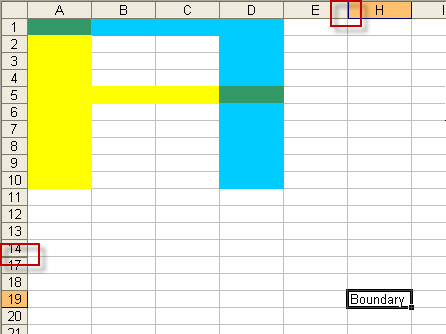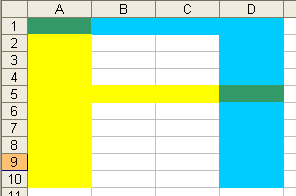# Adding a "Subtract Range" method alongside Union & Intersect

Published on
13,895 Points
6,495 Views
4 Endorsements
Last Modified:
Approved
Community Pick

## Introduction

Any VBA coder that has worked with Ranges will have made extensive use of the Intersect and Union Methods. Unfortunately VBA does not currently provide coders with a "negative join" option to remove one range from another.

This Article uses the approach from A fast method for determining the unlocked cell range to provide an efficient technique for subtracting ranges. Potential uses include:
1) Taking advantage of the efficiency of the SpecialCells Method to quickly derive other useful cell ranges, such as Hidden Cells = UsedRange - SpecialCells(xlVisible).
2) Excluding specific range areas prior to running other code, rather than running a continual exclusion test in the main code.
3) Combining the cells from two ranges together only where the cells do not overlap, effectively Union(rng1,rng2) - Intersect(rng1, rng2).

## Using the code

1. Copy the code at the bottom of this Article.
2. Open any workbook.
3. Press Alt + F11 to open the Visual Basic Editor (VBE).
4. From the Menu, choose Insert-Module.
5. Paste the code into the right-hand code window.
6. Close the VBE, save the file if desired.

In xl2003 go to Tools-Macro-Macros and double-click Test_the_Code.
In xl2007 click the Macros button in the Code group  of the Developer tab, then click Test_the_Codein the list box.

Please note that this code should be run from a regular VBA Code Module.

## The RemoveIntersect Function

This function accepts two ranges, removes the cells where the two ranges intersect, and then produces a string output containing the address of the reduced range. This is done by:
- creating a new one-sheet WorkBook,
- entering the N/A formula into all the cells on this sheet contained  in rng1,
- clearing the contents of all cells on this sheet that are contained by rng2,
- using SpecialCells to return the remaining N/A formulae which represents the cells in rng1 that are not found in rng2,
- If the Boolean variable, bBothRanges, is set to True, then the process is repeated with the cells with the opposite range order,
- the code then returns the "reduced" range as a string, then closes the WorkBook.

Note that the code uses a new one-sheet WorkBook for this example only, in practice if there were repeated Function calls it would make sense to use a specific WorkSheet as the working area rather than create a new WorkBook each time.  The WorkBook creation is redundant for the the first of the worked examples in this Article.

## The Test_the_Code Sub

This subroutine provides three worked examples consistent with the potential uses listed in the Introduction.
RangeIntersect.xls

In the first example there are hidden columns (F:G) and hidden rows (15:16) that intersect the UsedRange.The sample code test whether there are any hidden cells, if so then the RemoveIntersect function is used to returns the visible cell range as a string, by subtracting the visible cells within the UsedRange from the UsedRange. Note that the code explicity tests the UsedRange rather than simply ActiveSheet Cells, a sheet wide search would have taken longer processing time to return useless infomation outside the UsedRange.

The second and third examples work with two ranges.
- rng1 in A1:A10 and A5:D5
- rng2 in A1:D1 and D1:D10The second example removes any interesection of rng2 from rng1. In this case it removes cells A1 and D5.

The third example joins rng1 and rng2 together but removes the intersect in A1 and D5. The range join is accomplished by setting the optional Boolean bBothRanges argument to TRUE.

``````Function RemoveIntersect(ByRef rng1 As Range, ByRef rng2 As Range, Optional bBothRanges As Boolean) As String
Dim wb As Workbook
Dim ws1 As Worksheet
Dim rng3 As Range
Dim lCalc As Long

'disable screenupdating, event code and warning messages.
'set calculation to Manual
With Application
.ScreenUpdating = False
.EnableEvents = False
.DisplayAlerts = False
lCalc = .Calculation
.Calculation = xlCalculationManual
End With

'add a working WorkBook
Set wb = Workbooks.Add(1)
Set ws1 = wb.Sheets(1)

On Error Resume Next
ws1.Range(rng1.Address).Formula = "=NA()"
ws1.Range(rng2.Address).Formula = vbNullString
Set rng3 = ws1.Cells.SpecialCells(xlCellTypeFormulas, 16)
If bBothRanges Then
ws1.UsedRange.Cells.ClearContents
ws1.Range(rng2.Address).Formula = "=NA()"
ws1.Range(rng1.Address).Formula = vbNullString
Set rng3 = Union(rng3, ws1.Cells.SpecialCells(xlCellTypeFormulas, 16))
End If
On Error GoTo 0
If Not rng3 Is Nothing Then RemoveIntersect = rng3.Address(0, 0)

'Close the working file
wb.Close False
'cleanup user interface and settings
'reset calculation
With Application
.ScreenUpdating = True
.EnableEvents = True
.DisplayAlerts = True
lCalc = .Calculation
End With

End Function

Sub Test_the_Code()
Dim rngTest1 As Range
Dim rngTest2 As Range
Dim rngWorking As Range
Dim strTemp As String

'Example 1
'Return the hidden cell range on the ActiveSheet
Set rngTest1 = ActiveSheet.UsedRange.Cells
Set rngTest2 = ActiveSheet.UsedRange.SpecialCells(xlVisible)

If rngTest1.Cells.Count > rngTest2.Cells.Count Then
strTemp = RemoveIntersect(rngTest1, rngTest2)
MsgBox "Hidden cell range is " & strTemp, vbInformation
Else
MsgBox "No hidden cells", vbInformation
End If

Set rngTest1 = Range("A1:A10,A5:D5")
Set rngTest2 = Range("A1:D1,D1:D10")
Set rngWorking = Intersect(rngTest1, rngTest2)

'Example 2
'Remove any range overlap from rngTest1
If Not rngWorking Is Nothing Then
strTemp = RemoveIntersect(rngTest1, rngTest2)
MsgBox "Cells removed = " & rngWorking.Address(0, 0) & vbNewLine & _
"Remaining range is " & strTemp, vbInformation
Else
MsgBox "No overlap"
End If

'Example 3
'Combine rngTest1 and rngTest2 where the ranges do not overlap
'Uses the Boolean True argument to combine the ranges
If Not rngWorking Is Nothing Then
strTemp = RemoveIntersect(rngTest1, rngTest2, True)
MsgBox "Cells removed = " & rngWorking.Address(0, 0) & vbNewLine & _
"New combined range is " & strTemp, vbInformation
Else
MsgBox "No cell overlap" & vbNewLine & _
"New combined range is " & Union(rngTest1, rngTest2).Address(0, 0), vbInformation
End If
End Sub
``````

## Other Notes

Note that Excel supports a maximum of 8,192 non-contiguous cells through VBA macros. If the SpecialCells approach is applied to a selection that contains more than 8192 areas then the actions that were only supposed to occur with the non-contiguous cells occur to every cell in the selection. For more information see  The .SpecialCells(xlCellTypeBlanks) VBA function does not work as expected in Excel.

=-=-=-=-=-=-=-=-=-=-=-=-=-=-=-=-=-=-=-=-=-=-=-=-=-=-=-=-=-=-=-=-=-=-=-=-=-=-=-=-=
If you liked this article and want to see more from this author, please click here.

If you found this article helpful, please click the Yes button near the:

Was this article helpful?

label that is just below and to the right of this text.   Thanks!
=-=-=-=-=-=-=-=-=-=-=-=-=-=-=-=-=-=-=-=-=-=-=-=-=-=-=-=-=-=-=-=-=-=-=-=-=-=-=-=-=
4
Ask questions about what you read
If you have a question about something within an article, you can receive help directly from the article author. Experts Exchange article authors are available to answer questions and further the discussion.
Get 7 days free